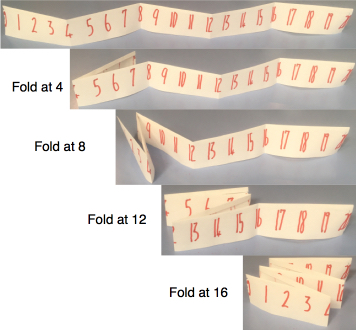#### You may also like### Prompt Cards

These two group activities use mathematical reasoning - one is numerical, one geometric.### Consecutive Numbers

An investigation involving adding and subtracting sets of consecutive numbers. Lots to find out, lots to explore.### Exploring Wild & Wonderful Number Patterns

EWWNP means Exploring Wild and Wonderful Number Patterns Created by Yourself! Investigate what happens if we create number patterns using some simple rules.

# Folded Number Line

## Folded Number Line

I made a number line from 0 to 20 and folded it as shown below:I folded it along the 4 and the 8 so that the 0 is on top of the 8 and the 4 is on top of the 12.

I then folded it along the 12 and the 16 so that now the 0 is on top of 8 which is on top of 16 and the 4 is on top of the 12 which is on top of the 20.

I ended up with some stacks of numbers that are on top of each other.

For example:
• 1, 7, 9, 15 and 17 make a stack which has total of 49.
• The stack made of 0, 8 and 16 has a total of 24.
• The stack made of 3, 5, 11, 13 and 19 has a total of 51.
We will be focusing on totals made in this way (like 49, 24 and 51 above).

### The challenges below involve varying the length of the number line. However, you must always start with 0 and each folded section must be the same length. There must always be at least one fold in the number line and folds must go through a number.

#### Challenge 1

Find three different ways to get a 'stack total' of 36 using lengths of 21 to 36 inclusive, showing exactly how you fold the number lines.

#### Challenge 2

Find as many ways as you can to get a 'stack total' of 48 using lengths of 21 to 36 inclusive, again showing exactly how you fold the number lines.

#### Challenge 3

Now using lengths from 21 to 50 inclusive, find a length which contains all the 'stack totals' of 69, 70 and 71 when folded.

This sheet contains a copy of the task.

### Why do this problem?

This task was designed for the 2018 National Young Mathematicians' Award, so it might be a particularly useful activity for a small group of your highest-attaining pupils to work on. It encourages a systematic approach and provides an opportunity for pupils to explain how they know they have all the solutions.

### Possible approach

Begin by showing children a paper number strip with numbers from 0-12 equally spaced. Fold the strip in half so that the 0 is on top of the 12, and talk about the pairs of numbers that are together. (In this case, stack totals all equal 12 except for the 6 which is on its own.)

Next, unfold the strip paper and re-fold it at 8 and 4. Discuss the phrase 'stack totals' - we want to know the totals of the numbers which are in a 'stack'. Here, the stacks are: 0 and 8; 4 and 12; 1, 7 and 9; 2, 6 and 10; 3, 5 and 11. Ask pupils to work out what the stack totals would be (these are 8, 16, 17, 18 and 19). Is there a pattern here? Why? Encourage pupils to look at how the numbers in each stack change as you move down the strip of folded paper.

Once pupils have understood how the folding and stack totals work, give them time to work in small groups on the three challenges.

### Key questions

Explain what you are doing to find the next solution.
Tell me about the stack totals you've got.
How are you trying to get a particular total?
Tell me about how you decide where to make the folds.

### Possible extension

Pupils could explore what happens to stack totals if the length of each fold can vary. For example, in the case of a 12 number line, you could have folded at 2 and 8. In this case you have 2 and 8 each on their own, then: 1 and 3; 11 and 5; 10 and 6; 9 and 7; 0, 4 and 12. In that case, the stack totals would be 2, 4, 16, 16, 16, 16 and 8.

### Possible support

Some pupils may need help in producing the number strips so that they can start the task. Providing lined or squared paper will help the children space the numbers equally on the strips.
Sharing different ways of thinking about the problem and different ways of recording that thinking can be very useful in developing all learners' problem-solving skills.